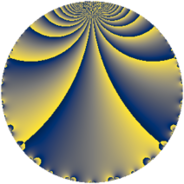# Properties

 Label 64.2.aLevel $64$ Weight $2$ Character orbit 64.a Rep. character $\chi_{64}(1,\cdot)$ Character field $\Q$ Dimension $1$ Newform subspaces $1$ Sturm bound $16$ Trace bound $0$

# Learn more about

## Defining parameters

 Level: $$N$$ $$=$$ $$64 = 2^{6}$$ Weight: $$k$$ $$=$$ $$2$$ Character orbit: $$[\chi]$$ $$=$$ 64.a (trivial) Character field: $$\Q$$ Newform subspaces: $$1$$ Sturm bound: $$16$$ Trace bound: $$0$$

## Dimensions

The following table gives the dimensions of various subspaces of $$M_{2}(\Gamma_0(64))$$.

Total New Old
Modular forms 14 3 11
Cusp forms 3 1 2
Eisenstein series 11 2 9

The following table gives the dimensions of the cuspidal new subspaces with specified eigenvalues for the Atkin-Lehner operators and the Fricke involution.

$$2$$Dim.
$$-$$$$1$$

## Trace form

 $$q + 2q^{5} - 3q^{9} + O(q^{10})$$ $$q + 2q^{5} - 3q^{9} - 6q^{13} + 2q^{17} - q^{25} + 10q^{29} + 2q^{37} + 10q^{41} - 6q^{45} - 7q^{49} - 14q^{53} + 10q^{61} - 12q^{65} - 6q^{73} + 9q^{81} + 4q^{85} + 10q^{89} + 18q^{97} + O(q^{100})$$

## Decomposition of $$S_{2}^{\mathrm{new}}(\Gamma_0(64))$$ into newform subspaces

Label Dim. $$A$$ Field CM Traces A-L signs $q$-expansion
$$a_2$$ $$a_3$$ $$a_5$$ $$a_7$$ 2
64.2.a.a $$1$$ $$0.511$$ $$\Q$$ $$\Q(\sqrt{-1})$$ $$0$$ $$0$$ $$2$$ $$0$$ $$-$$ $$q+2q^{5}-3q^{9}-6q^{13}+2q^{17}-q^{25}+\cdots$$

## Decomposition of $$S_{2}^{\mathrm{old}}(\Gamma_0(64))$$ into lower level spaces

$$S_{2}^{\mathrm{old}}(\Gamma_0(64)) \cong$$ $$S_{2}^{\mathrm{new}}(\Gamma_0(32))$$$$^{\oplus 2}$$